# Intersecting Lines And Non-intersecting Lines

Before talking about what intersecting lines and non-intersecting lines are, let us recall the basic definition of a line. In geometry, a line is something that is made up of infinite points extending indefinitely in both directions. It is straight and has negligible depth or width.  It is illustrated by little arrows on the two sides. A line has no ends. In case, if the line has an endpoint, then it is called a line segment.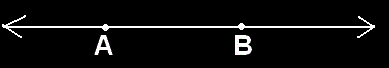Any two points can be taken and a line can be drawn which passes through them and extends in both directions. For example, the line in the figure above is denoted by

$$\begin{array}{l}\overleftrightarrow{AB}\end{array}$$
.

• Intersecting lines
• Non-intersecting lines

## Intersecting Lines

Two or more lines which share exactly one common point are called intersecting lines. This common point exists on all these lines and is called the point of intersection.  It is to be noted that:

• The intersecting lines meet at one, and only one point, no matter at what angle they meet.
• No two straight lines can meet at more than one point.
• The lines that meet at more than one point are not straight lines. At least one of them is a curve.

Let us take a few examples.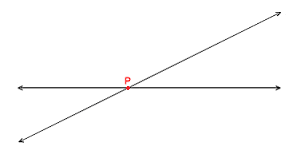Consider these lines. They are two intersecting lines. The point of intersection is P.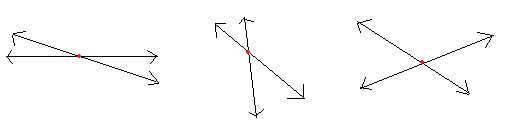The figure above also shows intersecting lines at different angles.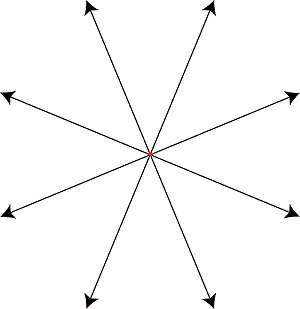The figure shows an example of 4 intersecting lines.

#### For More Information On Intersecting Lines and Parallel Lines, Watch The Below Video: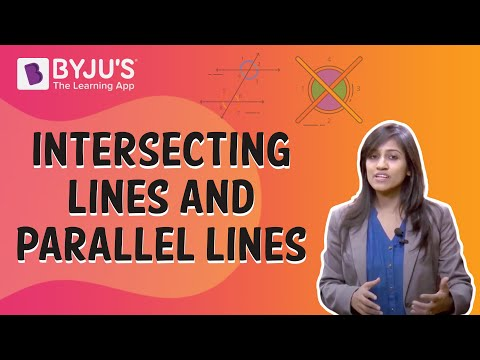## Non-intersecting Lines

Two or more lines that do not intersect each other are called non-intersecting lines. It is to be noted that:

• Non-intersecting lines can never meet.
• They are also known as the parallel lines.
• They are always at the same distance from one another. This is called the distance between two parallel lines.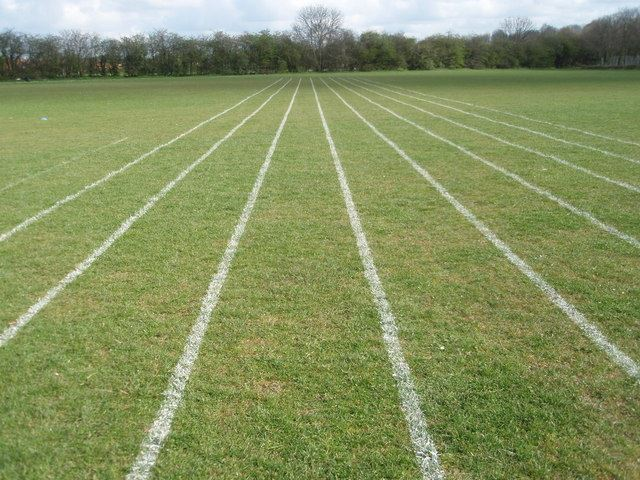The figures above show non-intersecting or parallel lines.